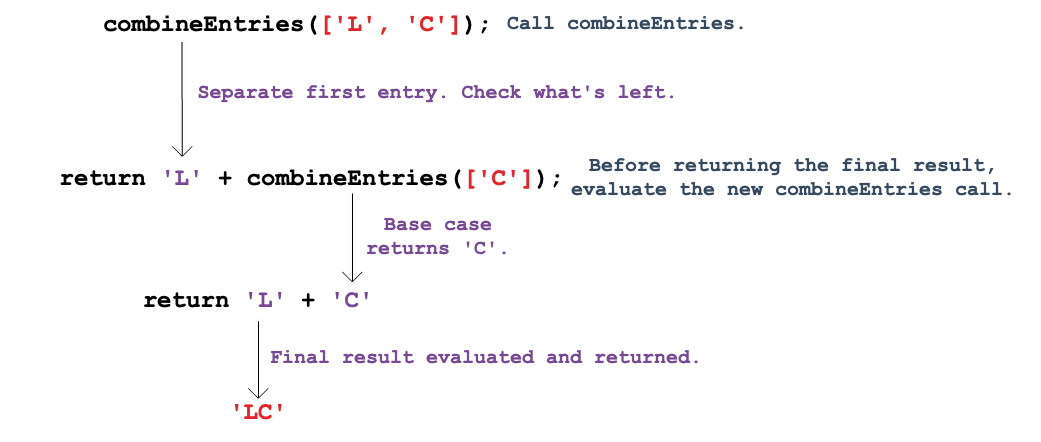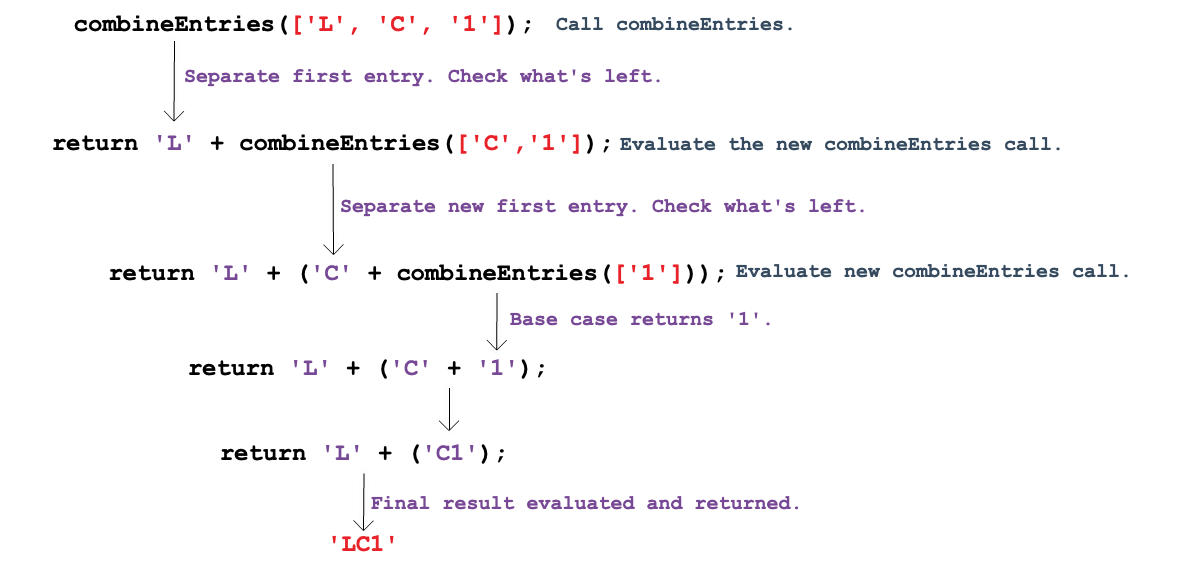# 11.8. Making A Function Call Itself¶

Congratulations! Identifying the base case is often the trickiest part of building a recursive function.

We've made it this far with `combineEntries`:

 ```1 2 3 4 5 6 7``` ```function combineEntries(arrayName){ if (arrayName.length <= 1){ return arrayName; } else { //call combineEntries again } } ```

Now we are ready to take the next step.

## 11.8.1. A Visual Representation¶

To help visualize what happens during recursion, let's start with the base case `['L']`:Nothing complicated here. `combineEntries` sees only one item in the array, so it returns `'L'`.

Now consider an array with two elements, `['L', 'C']`:In this case, `combineEntries` executes the `else` statement. We have no code for this yet, but we can still consider the logic:

1. `combineEntries` returns `'L'` and calls itself again using what is left inside the array (`['C']`).

2. When passed `['C']`, which is the base case, `combineEntries` returns `'C'`.

3. The strings `'L'` and `'C'` get combined and returned as the final result.

Next, consider an array with three elements `['L', 'C', '1']`:As before, `combineEntries` executes the `else` statement, and we can follow the logic:

1. `combineEntries` returns `'L'` and calls itself again using what is left inside the array (`['C', '1']`).

2. When passed `['C', '1']`, `combineEntries` returns `'C'` and calls itself again using what is left inside the array (`['1']`).

3. When passed `['1']`, which is the base case, `combineEntries` returns `'1'`.

4. The strings `'C'` and `'1'` get combined and returned.

5. The strings `'L'` and `'C1'` get combined and returned as the final result.

As we make the array longer, `combineEntries` calls itself more times. Each call evaluates a smaller and smaller section of the array until reaching the base case. This sets up a series of return events - each one selecting a single entry from the array. Rather than building `'LC101'` from left to right, recursion constructs the string starting with the base case and adding new characters to the front:

Value Returned

Description

`'1'`

Base case. Returns the element from an array of length 1.

`'01'`

Combines the first element from an array of length 2 with the base case value.

`'101'`

Combines the first element from an array of length 3 with the two previous values.

`'C101'`

Combines the first element from an array of length 4 with the three previous values.

`'LC101'`

Combines the first element from an array of length 5 with the four previous values.

Recursive processes all follow this approach. Each call to the function reduces a problem into a slighly smaller piece. The reduction continues until reaching the simplest possible form---the base case. The base case is then solved, and this creates a starting point for completing all of the previous steps.

## 11.8.2. A Function Calls Itself¶

So how do we code the `else` statement in `combineEntries`? Recall what needs to happen each time the statement runs:

1. Select the first element in the array,

2. Call `combineEntries` again with a smaller array.

Bracket notation takes care of part a: `arrayName`.

For part b, remember that the slice method returns selected entries from an array. To return everything BUT the first entry in `arr = ['L', 'C', '1', '0', '1']`, use `arr.slice(1)`.

Let's add the bracket notation and the `slice` method to our function:

 ```1 2 3 4 5 6 7``` ```function combineEntries(arrayName){ if (arrayName.length <= 1){ return arrayName; } else { return arrayName+combineEntries(arrayName.slice(1)); } } ```

Each time the `else` statement runs, it extracts the first element in the array with `arrayName`, then it calls itself with the remaining array elements (`arrayName.slice(1)`).

For `combineEntries(['L', 'C', '1', '0', '1']);`, the sequence would be:

Step

Description

1

First call: Combine `'L'` with `combineEntries(['C', '1', '0', '1'])`.

2

Second call: Combine `'C'`, with `combineEntries(['1', '0', '1'])`.

3

Third call: Combine `'1'`, with `combineEntries(['0', '1'])`.

4

Fourth call: Combine `'0'`, with `combineEntries(['1'])`.

5

Fifth call: Base case returns `'1'`.

To get the final result, proceed up the chain:

Step

Description

5

Return `'1'` to the fourth call.

4

Return `'01'` to the third call.

3

Return `'101'` to the second call.

2

Return `'C101'` to the first call.

1

Return `'LC101` as the final result.

Question

What if we wanted to take a number (n) and add it to all of the positive integers below it? For example, if n = 5, the function returns 5 + 4 + 3 + 2 + 1 = 15.

Consider the code sample below, which declares the `decreasingSum` function.

 ```1 2 3 4 5 6 7``` ```function decreasingSum(integer) { if (integer === 1){ return integer; } else { //call decreasingSum function again } } ```

Which of the following should be used in the `else` statement to recursively call `decreasingSum` and eventually return the correct answer?

1. `return integer + (integer-1);`

2. `return integer + (decreasingSum(integer));`

3. `return integer + (decreasingSum(integer-1));`

4. `return decreasingSum(integer-1);`

Experiment with this repl.it.